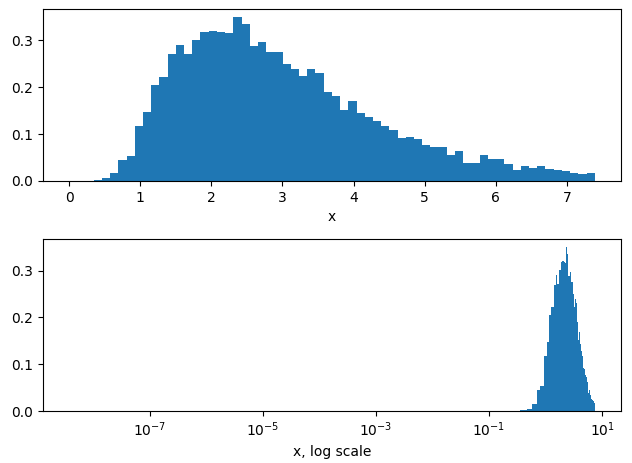# Use of LogNormal in Numpyro

Hi everyone,

I am very new to Numpyro and hierarchical modeling. Recently I have been trying to build a hierarchical model where I have a hyper-prior (theta_group) which should be centered around 1 and strictly positive. There is another prior (theta_part) which should be centered around theta_group. I am trying to use LogNormal as priors for both:

theta_group = numpyro.sample("theta_group", dist.LogNormal(1.0, 1.0))
tau_group = numpyro.sample("tau_group", dist.HalfCauchy(5))
theta_part = numpyro.sample("theta_part", dist.LogNormal(theta_group, tau_group))

• What should be the scale of the “mu” and “sigma” parameter for LogNormal. Am I declaring “theta_group” correctly?
• Also what is the scale of the output of LogNormal? Do I have to perform any transformation on “theta_group” or “tau_group” before using those for “theta_part”?

Thanks.

You’re a little off. In the LogNormal distribution, the loc and scale (aka \mu and \sigma) are the mean and standard deviation of the log of the parameter. I.e. if

x \sim \text{LogNormal}(5,1)

That means:

x = \exp(5 \pm 1)

The log normal distribution is when you have a rough idea of the scale of the parameter, but don’t much care about the specific number. In your example, you’d want to change to:

theta_group = numpyro.sample("theta_group", dist.LogNormal(1.0, 1.0))
tau_group = numpyro.sample("tau_group", dist.HalfCauchy(5))
theta_part = numpyro.sample("theta_part", dist.LogNormal(np.log(theta_group), tau_group))


That said, if you’re looking for “More or less normally distributed but strictly positive”, consider instead using distribution transforms to enforce x>0. These transforms, which look something like:


transformed_dist = dist.TransformedDistribution(
dist.Normal(mu, sig),
dist.transforms.SoftplusTransform(),
)

theta = numpyro.sample('theta', transformed_dist)


Can take a simple distribution like Normal and “squish” it into a more restrictive domain. This will only affect how the distribution looks around x\approx0, but leave it untouched in the rest of the domain.

2 Likes

Thanks a lot for your explanation and suggestions!

From my model I know that in the ideal case theta_group should be 1.0 in its original scale. Therefore, when defining the prior, I am trying to have a LogNormal distribution peaked at 1.0 with some flat standard deviation. However, dist.LogNormal(1.0, 1.0) should not make that kind of distribution. It would be more like dist.LogNormal(jnp.log(1.0), 1.0) right?

Also, for theta_part, I think I need to use theta_group without any transformation as it should be centered around jnp.log(jnp.exp(theta_group)). Did I get it right?

Seems about right to me. A good way to take the guesswork out is to just plot the log-normal distribution and tweak the parameters to see how they behave. There’s about a half dozen ways you could do this with various levels of complexity, but the quickest and easiest is to go:

Nsamples = int(1E4)
mu = 1
sig = 0.5
with numpyro.handlers.seed(rng_seed = 1):
X = numpyro.sample('x', numpyro.distributions.LogNormal(mu,sig), sample_shape=(Nsamples,))


The seed context forces random elements like the sample to behave more like regular non-stochastic variables by fixing a seed for the random number generator, letting us draw samples directly from the LogNormal distribution.

Then we just plot with plt.hist to see what sort of distribution we get, e.g.:


X = np.array(X) # Not necessary but seems to make plotting run faster on my computer

fig, ax = plt.subplots(2,1)
ax.hist(X, bins=64, range = (0, np.exp(mu+2*sig)), density=True)
ax.hist(X, bins=64, range = (0, np.exp(mu+2*sig)), density=True)
ax.set_xscale('log')

ax.set_xlabel("x")
ax.set_xlabel("x, log scale")
plt.tight_layout()
plt.show()You can see that the distribution peaks at x=\exp(\mu), where here \mu=1.

1 Like

Thanks for showing me an example. If I do the following modification of your code, then I see a peak at 1.0.

Nsamples = int(1E4)
mu = 1
sig = 0.5
with numpyro.handlers.seed(rng_seed = 1):
X = numpyro.sample('x', numpyro.distributions.LogNormal(np.log(mu)+sig**2,sig), sample_shape=(Nsamples,))


Now my question is how to define this part of my model:

theta_part = numpyro.sample("theta_part", dist.LogNormal(theta_group, tau_group))


Should I use dist.LogNormal(jnp.log(theta_group)+tau_group**2, tau_group) for theta_part?

Thanks.